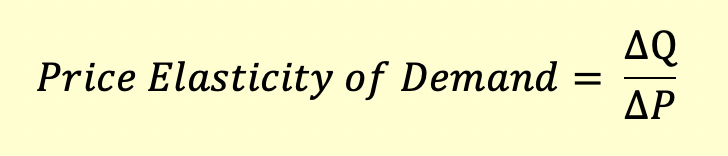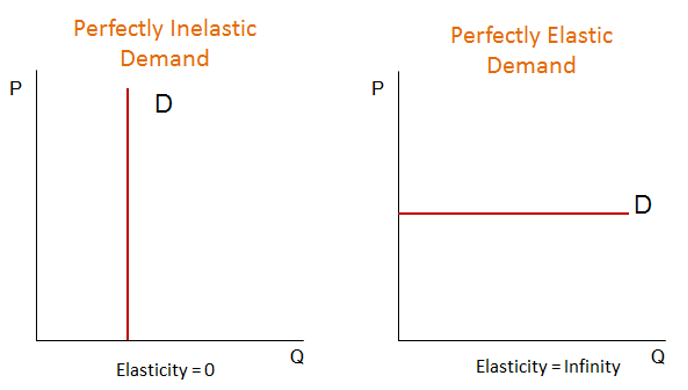# Price Elasticity of Demand

Price elasticity of demand is the most common form of elasticity. It measures the change in quantity demanded caused by a change in the price of the good. It’s calculated as a ratio of change in quantity demanded to change in price.• It measures the movement along the demand curve.
• It’s always negative and but expressed as an absolute value.
• Demand is said to be elastic when quantity demanded changes in response to change in price. If the quantity demanded is not very responsive to price change, it is said to be inelastic.
• A value greater than 1 indicates elasticity. % change in quantity demanded is greater than % change in price. A value between 0 and 1 indicates inelasticity. % change in quantity demanded is less than % change in price.

The following two graphs depict perfectly elastic and perfectly inelastic demand.A perfectly inelastic demand means that the demand for the good remains the same irrespective of a change in price. This means that the consumers have no alternative to purchasing the good. An example is a lifesaving drug for which people will be willing to pay any price. A change in price wouldn’t cause a change in quantity demanded.

When price elasticity is -1 it’s called unitary elasticity. This is the price/quantity combination that maximizes the total revenue (price * quantity).